# Fast.ai Lesson 10 notes — Part 2 v3

Source: Deep Learning on Medium

These notes are from Part 2 v3 lessons which are not released yet. Some of the material might not make sense without the notebooks and therefore I don’t recommend to read these if you are not in the course. I tried to add all things that weren’t clear from v2 or that didn’t exist then.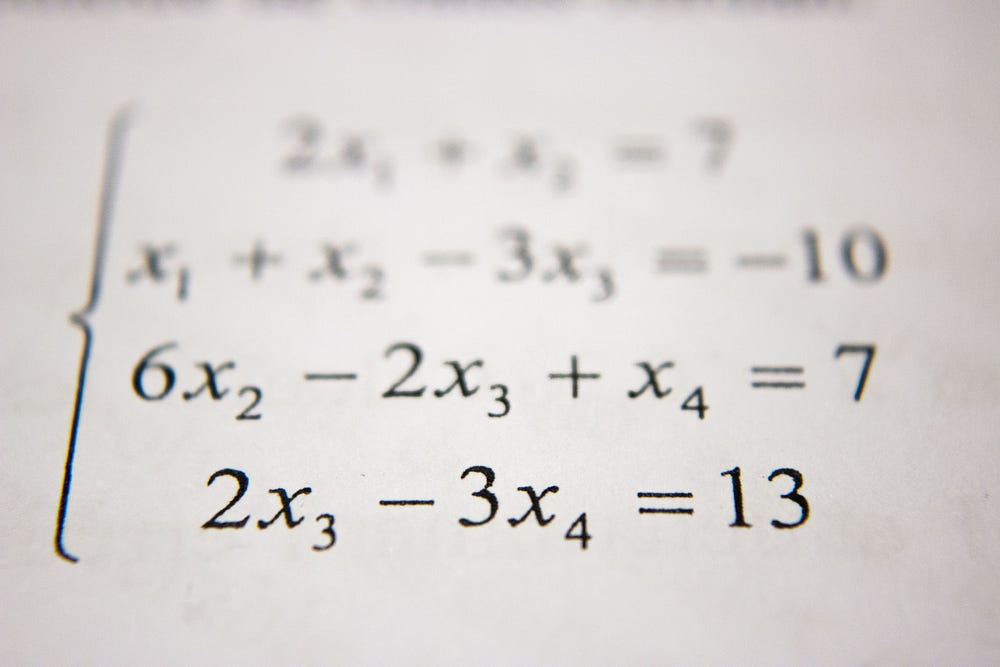A lot of math coming — Photo by Antoine Dautry on Unsplash

First I want to highlight one thing Jeremy said during the class. This phase might be really fast for most of the people and what he said is that this is not something he expects us to learn in 7 weeks but something he wants us to learn before he teaches another part 2 again next year. I try to write the most important things here but something I don’t want to do is to copy the full notebooks. Reading, running, and playing with those is an important thing to do and can’t be replaced with anything.

#### Prerequisite

`05a_foundations.ipynb`

Callbacks
Callbacks, as we have seen, are really useful for programmers and researchers. Last time I didn’t fully understand what callbacks are and I’m happy that Jeremy wanted to teach these more in details.

`import ipywidgets as widgets`
`def f(o): print('hi')w = widgets.Button(description='Click me')w.on_click(f)w`

The idea in `w.on_click(f)` is that we tell it that when button `w` is pressed callback to function `f`. As you can see we treat it like an object (there are no parentheses). Basically, callbacks are function pointers which means that we point the function to run. In the previous example we pointed out that function `f` need to be run when the button is clicked.

We just saw how callbacks work but how we can create our own callback? Let’s take an example.

As we can see the first version will just return the result in 5 seconds. Then we want to add a feature that tells the progress. `if cb: cb(i)` just checks if there is a callback and then it will call it. We create `show_progress` function that will take epoch number as input and then it prints a text. Now we can use `show_progress` as a callback and see the progress of calculation.

This is probably an easy example for everyone who is in this course. Jeremy showed this because we will learn much more complicated use cases for this thing. When you have a simple example it is easier to understand more complex things by comparing the differences.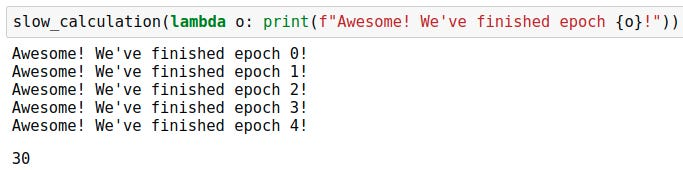We can define the function in place using lambda. You can think it as def but then you don’t add parentheses or name of the function.

In case our `show_progress` function includes two parameters but `cb(i)` includes only one we need a way to transform `show_progress` into one parameter function. That is happening below.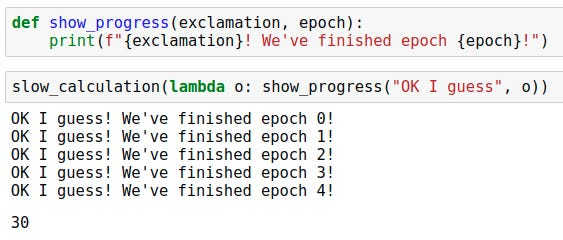This way we set the exclamation to always be “OK I guess”.

Maybe we want to make it easier to add different exclamations so we can just create a function for that.

Normally we want to do it like this:

This is called closure. It will return `_inner` function every time when `make_show_progress` is called.

This way we can make code like this:

Python already have the feature which make it easy to always use one variable value.

`"OK I guess"` will be used as first parameter in `show_progress` function so it become one parameter function.

Last week most of our codes used a class as a callback. So instead of using a closure (=function that returns a function) we can use a class.

`__init__` will be run when the object is created and `__call__` makes it plausible to use object same way as function.

`*args` and `**kwargs`One star will save all arguments as tuple when double star will save all arguments as dictionary.

`*args` positional arguments
`**kwar` key word arguments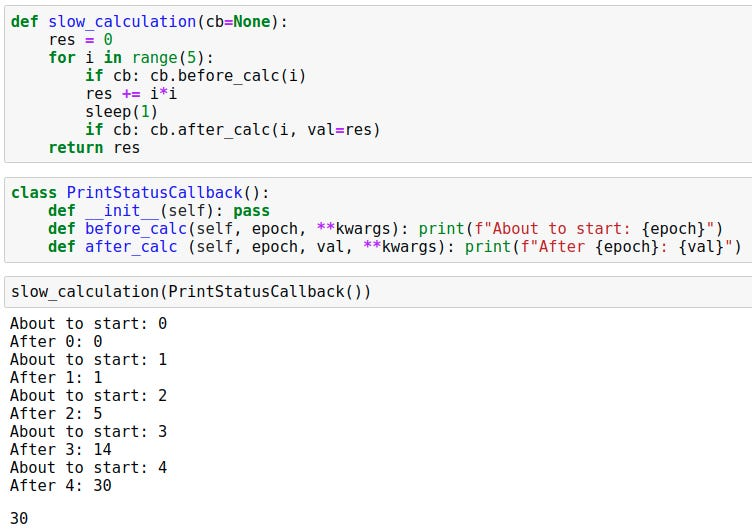We add **kwargs because maybe in the future we add some new parameters and we don’t want it to break this code.

This is great code and it starts to remind the code we used last time. Next, we want to add a couple of features.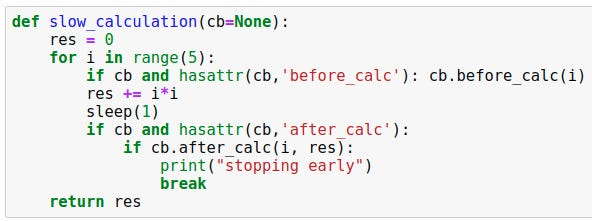Now in both of these cases, we first check is there this function we are going to call. Then in the latter case, we also want to add the ability to stop the code.

Then we can even change how the calculation is happening by defining it inside a function.

`__dunder__`
All variable names with a double underscore before and after are somehow special. You can read the meaning of all these from the Python documentation. You probably already have learned a few during this course.

Browsing source code — VIM examples

`:tag NAME_START` +tab — loop through different options that starts with `NAME_START` (E.g. `NAME_START_THIS`, `NAME_START_ANOTHER`)

click a function name and press ctrl + ] — go to the definition

ctrl + t — go back to the place where you came using above thing

`Ack lambda` — show all places where is lambda.

My own opinion about VIM is that it looks cool but it is not practical. Of course, using terminal editor looks more like you know something about coding but most of these things are just much more complicated than using GUI editor like VS Code. Some of the things can be learned but some will stay hard no matter how good you are using VIM.

Variance
Variance means how much data point varies which is same as how far they are from the mean.

The last two numbers tell us how far the data points are from the mean. `(t-m).pow(2).mean()` is called variance,`(t-m).pow(2).mean().sqrt()` is called standard deviation, and `(t-m).abs().mean()` is called mean absolute deviation.

Notice! `(t-m).abs().mean()` = `(t-m).pow(2).sqrt().mean()`

As you saw, the standard deviation is more sensitive and that is why we more often use absolute deviation. The only reason why mathematicians and statisticians use standard deviation instead of mean absolute deviation is that it makes the math easier.

`(t-m).pow(2).mean()` = `(t*t).mean() — (m*m)`

The bolded part is often easier to work with.

Covariance and correlation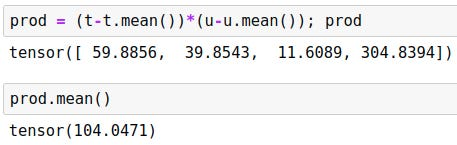prod is how far each point in x-axis is from the mean of the x-axis times how far each point in the y-axis is from the mean of the y-axis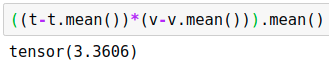The reason why this number is much smaller than above is that when data points set into line the x-axis increase same time with the y-axis. There will be two big positive or negative number multiplied with each other and the result is of course a big number.

This number tells how well points line up. It is called Covariance. These numbers can be at any range so we calculate correlation which is always between -1 and 1.

A great (3 min) video about the topic: https://youtu.be/85Ilb-89sjk

Softmax
We have learned many times how this thing works but this time Jeremy showed something that many people miss.

As you can see softmax vector for both of these images is identical although the output is different. The reason is that exp vector numbers are in the same ratio. Important about this is that although it looks like that there is a fish in both of images it might not be the case in the second image. In the first image, a fish caused the output to be about 2 but in the second picture, fish get’s output of 0.63. This means that there might not be any of these options in the picture. In the other hand, the same kind of problem can be caused if the first image contains cat, dog, and building. We can’t know which one of these happened but one thing we can be certain about is that the probabilities aren’t the same.

To summarize, softmax is a great metric if you have only (always) one plausible item in the image. If there is none it still chooses one or if there is multiple it need to choose only one.

Multi-label binary classification is better for this. Instead of dividing the exp number with sum of these number you divide it with 1+exp.

In real world, binary loss is better because most of the cases your data includes images with multiple items or images without items.

#### Using exception as a way to stop code

`05b_early_stopping.ipynb`

We need a way to stop the fit function at some point. When we look at the code we made last time, we notice that in available to stop it, we need to return true in a lot of different callbacks which makes the code complicated. Jeremy suggested this idea of using exceptions as a way to stop the code.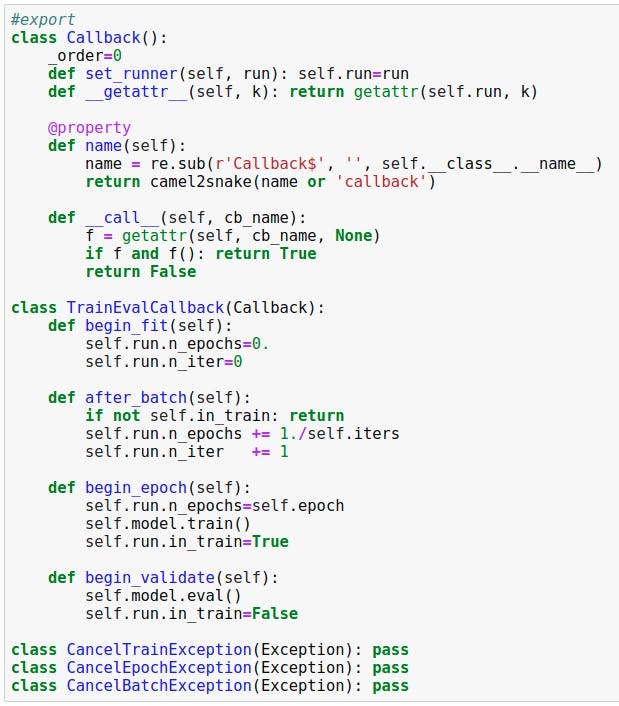There is again some refactoring from the last time. We have moved the callback usage from runner to callback itself. This again adds more flexibility.

In the end, there are three lines of code that are important to notice. These are our own custom exceptions. In Python own exception can be created by inheriting `Exception` class. The idea of these exceptions is to make it plausible to cancel train, epoch, or batch.

Notice that now there is try — except — finally added around the code. If an exception happens there is after exception callback and then it stops and in other cases, it runs normally. There is no error or any other message (if we don’t want) when we use exception.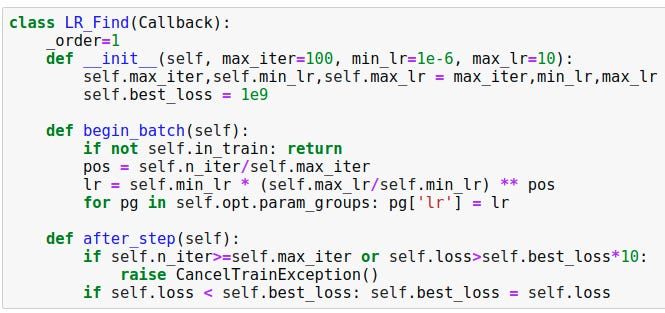Learning rate finder using the exception method. This is not currently in Fast.ai 1.0 but it will be added to the later version.

#### ConvNet

`06_cuda_cnn_hooks_init.ipynb`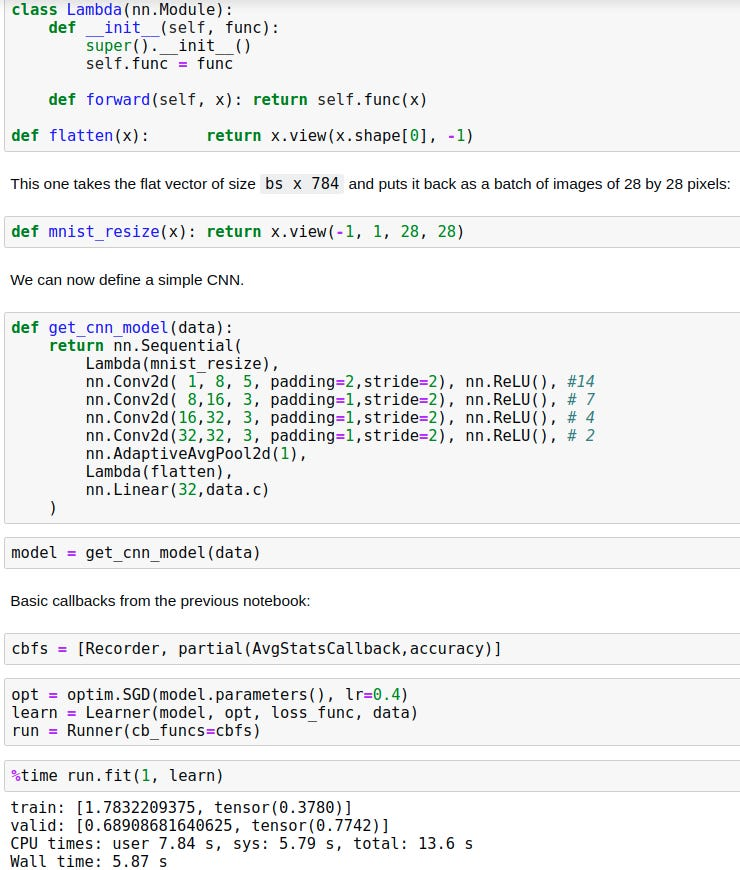First just basic ConvNet. As you can see the model is slow and to speed it up we want to use GPU.

To use a GPU for calculation we need to put the model’s parameters and the inputs into a GPU. Let’s create a callback for this.

After fit and batch, we move the model’s parameters and inputs into the GPU we have.

First I thought that I’m not going to add the following screenshot here because it is again the same thing with callbacks but then Jeremy pointed that this is a great example of partial and other things we have learned this lesson.

#### Hooks

The idea of hooks is that we want to see what is happening inside the model when we train it.

In above example we want to see how the mean and standard deviation changes. We save those to the lists between the layers.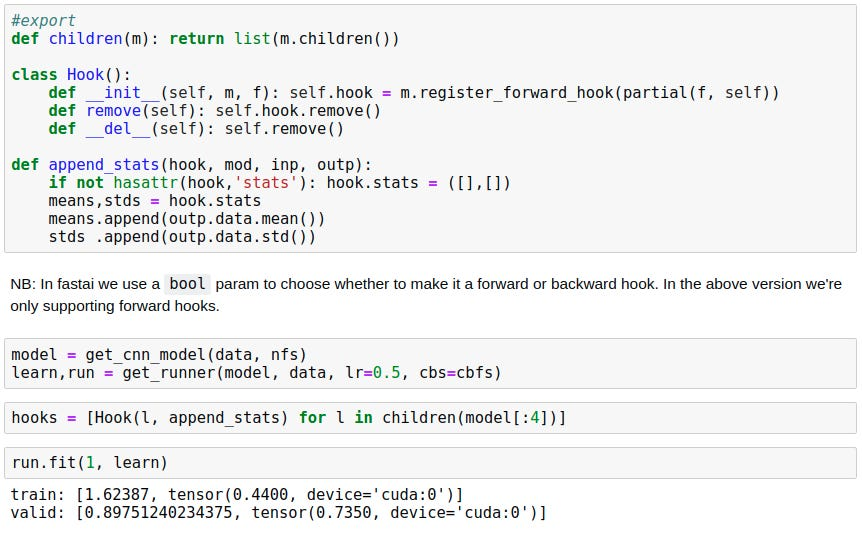We can refactor the thing above to look like this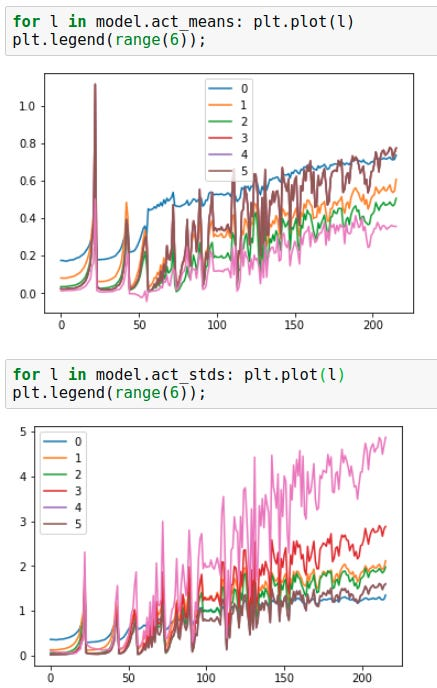We can plot those lists and see better what is happening these values.

As you can see from the plots these values increases exponentially and collapse suddenly many times at the start. Then later the values stay better at some range which means that model starts to train. If these values just goes up and down model is not learning anything.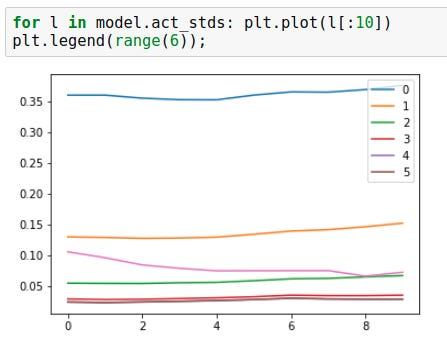When we look at the first ten loops we notice that the standard deviation drops further we go from the first layer. And to recall we wanted this to be close to one.

We solve above problem using kaiming initialization.

After this the plots looks much better.

One thing we want to assure is that the activations aren’t really small. This can be seen using histogram.

These plots show us right away a problem. There is a yellow line going bottom of each plot and that means there is a lot of values and that is something we don’t want.

The plots above show how many percentages of activations are nearly zero. This tells us more about the yellow line we saw on previous plots.

To make this better we want to test a different kind of ReLUs.

With these…

`model = get_cnn_model(data, nfs, conv_layer, leak=0.1, sub=0.4, maxv=6.)`

…hyperparameters, we get a nice plot that looks more like what we want.

Now we are using most of our activations by initializing the values well and paying attention to the ReLU function.

In this notebook there were a lot of small software engineering things that are good to go though so instead of just copying the full notebook here I want you to open that and test it. Go through the code and if there is something you don’t understand watch the video again.

#### BatchNorm

`07_batchnorm.ipynb`

We have now learned how to initialize the values to get better results. We have reached our limit and to go further we need to use normalization. BatchNorm is probably the most common normalization method.

In math, we use gamma and beta but in the code we use words `mults` and `adds`. These are first initialized into ones and zeros but because these are parameters we will learn them. The different thing we have is that instead of using mean and variance of the batch for training we use a running average. We calculate the running average following way. First we set variances and means using buffers `self.register_buffer('vars', torch.ones(1,nf,1,1))`. This is works like `self.vars = torch.ones(1,nf,1,1)` except it moves the values to GPU when the whole model is moved. Also, we need to store these values the same way we store other parameters. This will save the numbers when the model is saved.

Normally running (or moving) average is calculated (picture above) by taking n points and calculating the average of those. Then if we get a new point we will drop the last point of old batch and calculate the average for that batch. This time we don’t want to use this because we might have hundreds of millions of parameters which means that calculations can take a lot of time.

We use instead of this calculation. We take most of the old value and add a little bit of new value to it. This is called linear interpolation (in Python `_lerp`). This way further we go less the first values will have an effect. The effect actually decreases exponentially. This way we need to keep only track of one number.

If we use BatchNorm we don’t need bias because there is already a bias in BatchNorm.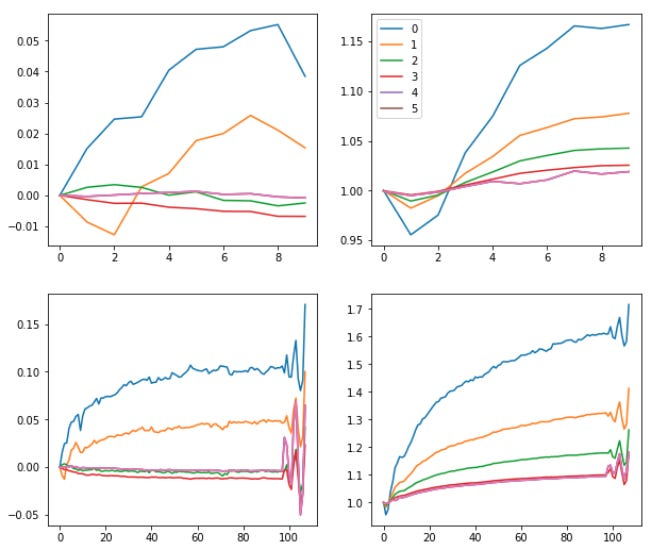After BatchNorm we see how well our standard deviation and mean changes.

#### More norms

BatchNorm works well in most of the cases but it cannot be applied to online learning tasks. Online learning means that we learn after every item. For example, robot running. We want to train it the same time when it is running so we train it after every step. The problem is that the variance of one data point is infinite. This problem can happen actually even more than one point if all of those are the same values. So to generalize better we can say that BatchNorm is not good for small batch sizes. Another problem is the RNNs. We can’t use BatchNorm with RNNs and small batches.

LayerNorm is just like BatchNorm except instead of `(0,2,3)` we have `(1,2,3)` and this doesn’t use the running average. It is not even nearly as good as BatchNorm but for RNNs it is something we want to use because we can’t use BatchNorm.

The problem with LayerNorm is that it combines all channels into one. InstanceNorm is a better version of LayerNorm where channels aren’t combined together.

This is not for image classification but for style transfer. This was just an example of how to not choose the normalization. You need to understand what it is doing in available to understand is it something that might work. Maybe you could just test but if you don’t have any idea what it is doing it may cause other kinds of problems later which are hard to debug.

Picture above shows us how BatchNorm takes the mean and variance over all batches. Then LayerNorm takes the mean and variance over all of the channels. InstanceNorm takes the mean and variance of one batch and one channel. GroupNorm takes a random group of channels in one batch and calculate the mean and variance of those.

#### Fix small batch size problem

Jeremy said that he doesn’t have a solution for the RNN problem but he has a solution to the small batch size problem that BatchNorm has.

Mathematicians and programmers often use epsilon to describe some small number. One thing we might want to use this is for a division. If the denominator (number below the line) is a very small number computer might not be available to calculate it without adding epsilon.

If we use much higher epsilon value (e.g. 0.1) in BatchNorm it will assure that values can’t be very big. Another solution Jeremy had was RunningBatchNorm.

The idea in RunningBatchNorm is that we don’t use the mean or standard deviation of the batch but instead the running average version. This works because, for example, with batch size of two sometimes these numbers happen to be the same but when we have this running average we don’t care about the numbers being the same.

There is a few small details that need to be fixed and we will learn more about these later. This is just quick introduction to all of these and it is not important to remember everything right away.

1. We can’t take the running average of variances. It is just stupid because it doesn’t mean anything and batch sizes can change. We learned previously (picture below) that we only need to keep track of sums of squares of the data points and the sums of the data points. This way we can calculate the real variance just storing two values.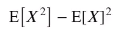We learned previously that we can calculate the variance this way.

2. Because the batch size can change it is important to also keep track of that too. Then we just divide the mean and variance with this value.

3. We need to do debiasing. It means that we don’t want any observation to be weighted too highly. The problem is that the first value will have too much power when it is in all of the calculations. This can be solved just by initializing the first values to zero.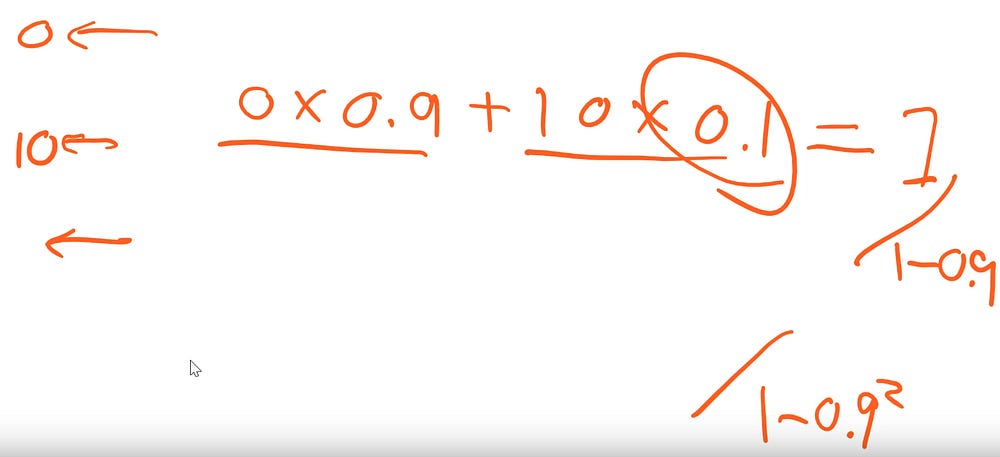If we start with the zero we can just divide the result with 1–0.9^n where n is number of numbers we have in running average.

~Lankinen